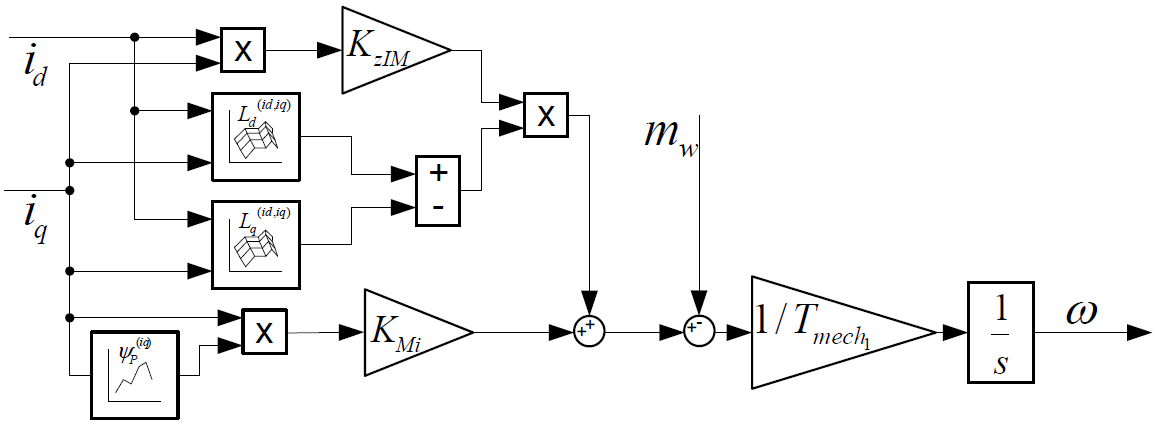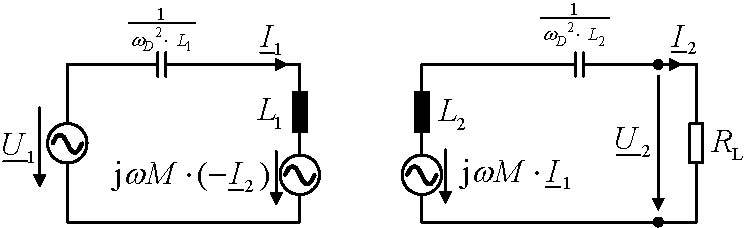# Analytical Modelling

Investigation of different analytical approaches for a more detailed description of electrical machines and contactless energy transmission systems.

### Calculation and Modeling

A central part of the basic research at IEW is the calculation and modelling of the electromagnetic energy converters developed here in various degrees of abstraction and detail. In addition to the use of such equation-based models for a fast and efficient optimization and design of our components and for overall system simulations in various operating points, the scientific analysis and improved understanding of interrelationships and influencing variables are also in the foreground.

### Analytical field calculation

For simple or idealized coil arrangements, the magnetic field can be resolved using the Biot-Savart attachment. Particularly long line conductors and ring-shaped coils can thus be calculated very quickly. The field of two coupled coils and important design parameters such as the coupling factor can also be determined quickly and efficiently. This method is used to design the coil geometry for inductive energy transmission systems.

Another approach is the direct solution of the Laplace or Poisson differential equation for certain 2D field problems by separating the variables. The solution is a series with an infinite number of summands, which is why the calculation in the MATLAB script is terminated after n iterations. Thus it is not an analytically closed solution, but the series converges very quickly and the error is therefore very small. This method is particularly suitable for brushless DC motors and synchronous motors, for example to investigate various winding schemes and their effects on torque ripple.

### Magnetic Equivalent Circuit and Gyrator Capacity Model

The modeling of flux paths by setting up a magnetically equivalent cuircit (MEC) and its solution with the aid of the network theory known from circuit technology enables a fast prediction of the electromagnetic properties of a magnetic circuit. It is also possible to take saturation and iron losses into account. The coupling and interaction between the magnetic and electrical circuits can be considered by a so-called gyrator. This allows simulations of a magnetic component and its control.

## Control Models

To design a suitable control system, a System model of the controlled system is required. This can be either linearized or based on maps from measurements, simulations or other calculations. The modeling is done in the Laplace frequency range. Electrical machines are typically temporary non-linear multi-variable systems whose parameters can usually not or only indirectly be determined in measurements. Based on this, standardized models such as the state space model can be derived in control engineering and controllers, observers and parameter estimation methods can be adapted accordingly.Shown is a block diagram for the force calculation of an electric machine with field-oriented control

### System modeling of inductive transmission paths

To model the system behavior of inductive transmission lines, individual parts of the entire power path are, as far as possible, considered separately and converted into an equivalent AC replacement model. The main parts are the primary-side power electronic circuit (represented by an equivalent AC or AC voltage source), the secondary-side rectifier circuit including load (represented by an equivalent load resistance), and the system of coils and compensation capacitors itself. These models can be used to determine the basic system behavior. This knowledge of system behavior is an essential basis for a structured system design.The figure shows the equivalent circuit diagram of a contactless power transmission link with serial compensation.